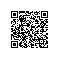# JavaScript权威指南 - 数组

JavaScript数组是一种特殊类型的对象。
JavaScript数组元素可以为任意类型，最大容纳232-1个元素。
JavaScript数组是动态的，有新元素添加时，自动更新length属性。
JavaScript数组元素索引可以是不连续的，它们之间可以有空缺。

## 创建数组

var a = new Array();            //空数组，等同于数组直接量[]
var b = new Array(5);           //创建指定长度的数组
var c = new Array(1, 5, 9, 6);  //指定一个或多个元素的非空数组

var a = [];                     //空数组
var b = [2, 3, 5, 7];           //常规元素
var c = [5, true, "a"];         //元素不同类型的数组
var d = [{ x: 2 }, { x: 3 }];   //包含对象元素的数组

var e = [1, , 3]; //该数组有3个元素，中间的元素为undefined
var f = [1, 5, ]; //该数组有2个元素，结尾逗号后面没有元素

## 数组元素

var arr = ["one", "two"];
var res = arr;  //读第0的元素
arr = "test";   //写第0的元素

var obj = [1, 2, 3];
obj["IsShow"] = false;   //现在obj数组为 [1, 2, 3, IsShow: false]

## 稀疏数组

var a = new Array(5); //数组没有元素，但a.length等于5
var b = [];
b = 1000; //添加一个索引为1000的元素，但b.length等于1001

## 数组长度

['a', 'b', 'c'].length; //最大索引为2，length为3

var a = [1, 2, 3, 4, 5]; //数组初始化5个元素
a.length = 3;  //现在a为[1,2,3]
a.length = 0;  //删除所有元素，a为[]
a.length = 5;  //数组长度为5，但是没有元素

var b = [1, 2, 3];
Object.defineProperty(b, "length", { writable: false }); //让length变成只读属性
b.length = 0; //更改无效

## 数组遍历

var obj = { height: 175, weight: 60 }; //初始化一个对象
var keys = Object.keys(obj);           //获取对象obj属性名组成的数组
var values = [];                       //values用来保存对象obj属性值
for (var i = 0, len = keys.length; i < len; i++) {
var key = keys[i];                 //获取当前索引的键值
values[i] = obj[key];              //在values数组中保存属性值
}

for (var i = 0; i < arr.length; i++) {
if (!arr[i]) continue;  //跳过null,undefined和不存在的元素
if (arr[i] === undefined) continue; //跳过undefined和不存在的元素
if (!(i in arr)) continue; //跳过不存在的元素
//T0DO
}

## 多维数组

JavaScript不支持真正的多维数组，一般用数组的数组来近似。下面是一个具体的例子，使用二维数组作为一个9X9乘法表。

//创建一个多维数组
var table = new Array(10); //表格有10行
for (var i = 0; i < table.length; i++) {
table[i] = new Array(10); //每行有10列
}
//初始化数组
for (var row = 0; row < table.length; row++) {
for (var col = 0; col < table[row].length; col++) {
table[row][col] = row * col;
}
}
//使用多维数组来计算
var result = table; //result = 72

## 数组方法

ECMAScript 3在Array.prototype中定义了一些很有用的操作数组的方法，下面介绍这些方法的基本用法。

join()
Array.join(separator)该方法可以将数组元素按照指定字符连接起来，返回最终生成的字符串。如果不指定字符separator，默认用逗号分隔。

var arr = [1, 2, 3];
arr.join();     //=>"1,2,3" 默认使用逗号作为元素连接符
arr.join(' ');  //=>"1 2 3" 以空格作为连接符
arr.join('|');  //=>"1|2|3" 以‘|’作为连接符

reverse()
Array.reverse()该方法将数组中的的元素颠倒顺序，在原数组上进行操作。方法返回值为对原来数组的引用。

var arr = [1, 2, 3];
arr.reverse().join(); //=>"3,2,1" ,并且现在arr为[3,2,1]

sort()
Array.sort([compareFunction])该方法将数组中的元素排序并返回对原来数组的引用。不传递参数调用时，默认按照字母顺序排序。

var fruits = ['banana', 'cherry', 'apple'];
fruits.sort().join();  //=>apple,banana,cherry

• 若 a 小于 b，在排序后的数组中 a 应该出现在 b 之前，则返回一个小于 0 的值。
• 若 a 等于 b，则返回 0。
• 若 a 大于 b，则返回一个大于 0 的值。
var s = [33, 666, 12, 5];
s.sort();                 //字母顺序：12,33,5,666
s.sort(function (a, b) {  //数字顺序：5,12,33,666
return a - b;
});

concat()
Array.concat(arr1[,arr2,...])该方法用于连接两个或多个数组并返回一个新数组，不会改变现有数组本身。

var a = [1, 2];
a.concat(4, 5);           //=>[1,2,4,5]     连接每一个参数值
a.concat([4, 5]);         //=>[1,2,4,5]     连接一个数组
a.concat([4, 5], [6, 7]); //=>[1,2,4,5,6,7] 连接多个数组 

slice()
Array.slice(start[,end])该方法用来从已有的数组返回选定的元素，返回一个新的数组。两个参数分别指定要选定元素的开始位置和结束位置。

• start参数表示从什么位置开始取。如果是负数，那么它规定从数组尾部开始算起的位置。也就是说，-1 指最后一个元素，-2 指倒数第二个元素，以此类推。
• end是一个可选参数。规定从何处结束选取，但不包括该下标元素。如果没有指定该参数，那么切分的数组包含从 start 到数组结束的所有元素。如果这个参数是负数，那么它规定的是从数组尾部开始算起的元素。
var a = [1, 2, 3, 4, 5];
a.slice(0, 3);     //返回[1,2,3]
a.slice(3);        //返回[4,5]
a.slice(1, -1);    //返回[2,3,4]
a.slice(-3, -2);   //返回

splice()
Array.splice(index,count[, item1[, item2[, ...]]])该方法用来向数组中添加或删除元素，并且用参数列表中声明的一个或多个值来替换那些被删除的元素。返回被删除的元素。该方法会改变原始数组。

• index参数代表要添加或删除元素的索引。
• count参数代表要从数组中删除的元素个数。如果省略，从index起点到数组结尾的元素全删除。
• item1,item2,...从第三个参数开始是可选参数。代表向数组添加的新元素。
var names = ["George", "Thomas"];
names.splice(1, 0, "John");  //在索引为1的地方插入一个新元素
names.splice(0, 1, "Tom");   //将第一个元素'George'替换成'Tom'

splice()slice()方法，一个字母之差但是功能完全不同，注意区别使用。

push()和pop()
Array.push(element1,element2,...)该方法用来向数组的末尾添加一个或多个元素，并返回新的长度。
Array.pop()方法用来删除数组的最后一个元素，减小数组长度，返回删除的元素值。

var stack = [];      //空栈
stack.push(1, 2);    //stack：[1,2]
stack.pop();         //stack：
stack.push(3);       //stack：[1,3]
stack.pop();         //stack：
stack.push([4, 5]);  //stack：[1,[4, 5]]
stack.pop();         //stack：
stack.pop();         //stack：[]

unshift()和shift()
Array.unshift(element1,element2,...)该方法可向数组的开头添加一个或更多元素，并返回新的长度。
Array.shift()方法用于把数组的第一个元素从其中删除，并返回第一个元素的值。如果数组为空，shift()不进行任何操作，返回undefined

var arr = [3, 4];
arr.unshift(1,2);   // arr：[1,2,3,4]
arr.shift();        // arr：[2,3,4] 

toString()和toLocalString()

[1, 2, 3].toString();        //"1,2,3"
["a", "b", "c"].toString();  //"a,b,c"
[1, [2, 'c']].toString();    //"1,2,c"

toLocalString()toString()的本地化方法。

ECMAScript 5中定义了9个新的数组方法来遍历，映射，过滤，检测，简化和搜索数组。有了这些方法就不用利用for循环来遍历数组了。

forEach()
Array.forEach(callback[, thisArg])方法用来从头致尾遍历数组，为每个元素调用回调方法。对于稀疏数组，不存在的元素不调用回调方法。

• callback参数就是在数组每一项上执行的函数，接收三个参数：数组元素、元素索引和数组本身。
• thisArg是可选参数，用来当作callback函数内this的值的对象。如果省略了thisArg参数,或者赋值为nullundefined，则 this 在非严格模式下将是全局对象，严格模式下为 undefined

var numbers = [5, 6];  // Define an array.
var obj = {
showResults: function(value, index,array) {
var squared = this.calcSquare(value);
document.write("value: " + value);
document.write(" index: " + index);
document.write(" squared: " + squared);
document.write("<br />");
},
calcSquare: function(x) { return x * x }
};
numbers.forEach(function(value, index) { this.showResults(value, index) }, obj);
// Output:
//  value: 5 index: 0 squared: 25
//  value: 6 index: 1 squared: 36

map()
Array.map(callback[, thisArg])方法和forEach()同样是用来遍历数组，为每个元素执行回调方法。该方法参数与forEach()方法参数一致，不再赘述。但是传给map()的函数应该有返回值。

var numbers = [1, 4, 9];
var roots = numbers.map(Math.sqrt); //求数组中每个元素的平方根
/* roots的值为[1, 2, 3], numbers的值仍为[1, 4, 9] */

filter()
Array.filter(callback[, thisArg])方法用来过滤数组元素，将符合规则的元素组成一个新数组返回，不会改变原数组。callback参数就是用来测试数组中元素的方法，返回true表示通过测试。

var arr = [1, 2, 3, 4, 5];
var res = arr.filter(function (value, index, array) {
return value > 3;  //过滤掉小于等于3的元素
});
alert(res.toString()); //=> 4,5

arr.filter(function (value) { return value != undefined && value != null; });

every()和some()
Array.every(callback[, thisArg])该方法用来测试数组元素是否都通过了指定函数的测试。
Array.some(callback[, thisArg])该方法用来测试数组某些元素是否通过了指定函数的测试。

var arr = [1, 2, 3, 4, 5];
arr.every(function (value) { return value < 10; }); //=>true 所有元素值<10
arr.every(function (value) { return value % 2 == 0; }); //false 并非所有元素都为偶数
arr.some(function (value) { return value % 2 == 0; }); //=>true 数组元素包含偶数
arr.some(isNaN); //=>false 数组不包含非数值元素

reduce()和reduceRight()
Array.reduce(callback[, initialValue])该方法会针对数组中每个元素调用指定回调函数，将回调函数的返回值作为累积，然后以参数的形式传递到下个元素的回调方法中。

• callback参数是数组元素要执行的回调函数。最多可接收4个参数：之前元素累积值、当前元素值、元素索引、数组本身。
• initialValue是可选参数，表示元素最开始调用回调函数传入的初始值。如果缺省该参数，它会使用数组第一个元素作为初始值，这样数组就会少迭代一次。
var arr = [1, 2, 3, 4];
var sum = arr.reduce(function (previous, current, index, array) {
return previous + current;
}, 5);
var max = arr.reduce(function (previous, current, index, array) {
return previous > current ? previous : current;
});
console.log(sum); //=>15  求和
console.log(max); //=>4   求最大值

var matrix = [ [1, 2], [3, 4], [5, 6] ];
var flatten = matrix.reduce(function (previous, current) {
return previous.concat(current);
});
console.log(flatten); //=> [1, 2, 3, 4, 5, 6]

Array.reduceRight(callback[, initialvalue])方法的用法与reduce()方法一致，唯一区别是该方法按元素索引降序处理元素。

indexOf()和lastIndexOf()
Array.indexOf(searchvalue[, fromIndex])方法用来搜索数组中给定值的元素，并返回该元素的索引，如果找不到指定的元素则返回-1。indexOf()从数组头至尾开始搜，Array.lastIndexOf(searchvalue[, fromIndex])则相反，从数组尾部为起点开始搜。

• searchvalue参数代表要搜索的元素值。
• fromindex是可选参数，表示检索的起始位置。其值可以为字符串数值；填入字符自动忽略，默认为0。
var data = [2, 5, 7, 3, 5];
console.log(data.indexOf(5, "x")); //=> 1 "x"被忽略,用0代替
console.log(data.indexOf(5, "3")); //=> 4  从3号位开始搜索

if (!Array.prototype.forEach) {
Array.prototype.forEach = function (callback, thisArg) {
//TODO
};
}

## 数组类型

console.log(Array.isArray([])); //=> true
console.log(Array.isArray({})); //=> false

instanceof操作符可以检测，但有局限性，只能作用于单页面的情形。

console.log([] instanceof Array);  //=> true
console.log({} instanceof Array);  //=> false

ECMAScript 3版本中，检测对象是否为数组的isArray()可以这样写：

var isArray = Array.isArray() || function isArray(arg) {
return typeof arg === 'object' &&  //是否为对象
object.length === 'number' &&  //验证length属性
Object.prototype.toString.call(arg) === '[object Array]'; //判断基本类型*
}

## 类数组对象

//定义一个类数组对象
var obj = { 0: 'a', 1: 'b', 2: 'c', length: 3 };
//当作数组遍历
for (var i = 0; i < obj.length; i++) {
console.log(obj[i]);
}

JavaScript函数体中Arguments对象是一个类数组对象。一些DOM方法也返回类数组对象，比如
document.getElementsByTagName()

function isArrayLike(o) {
if (o &&                                //判断o非null，undefined等
typeof o === 'object' &&            //o是对象
isFinite(o.length) &&               //o.length是有限数
o.length > 0 &&                     //o.length是非负数
o.length < 4294967296 &&            //o.length小于2^32
o.length === Math.floor(o.length))  //o.length是整数
return true;
else
return false;
}

ES5版本中，所有Array数组方法都是通用的，类数组对象上同样适用。类数组对象没有继承至Array.prototype不能直接调用，但可以通过Function.call方法调用：

var obj = { 0: 'a', 1: 'b', 2: 'c', length: 3 };
console.log(Array.prototype.join.call(obj, '|'));  //=> a|b|c
var arr = Array.prototype.map.call(obj, function (value) {
return value.toUpperCase();
});
console.log(arr.join); //=> A,B,C   此时arr已是真正的数组

## 参考与扩展

 David Flanagan，JavaScript权威指南(第6版)
 MDN，JavaScript 参考文档 - Array - JavaScript | MDN使用钉钉扫一扫加入圈子
+ 订阅